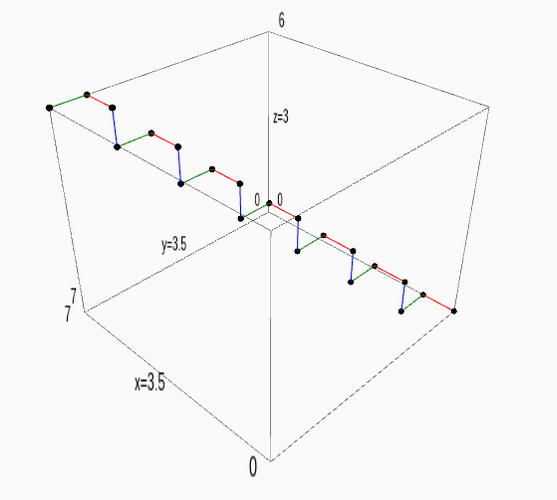# Revision history [back]

Maybe you can try something of the form

pts=[[1,2,3],[1,1,1],[2,3,0]]
line3d(pts,color='red')+point3d(pts,color='blue')


You will probably need to plot each of the line segments separately.

Maybe you can try something of the form

pts=[[1,2,3],[1,1,1],[2,3,0]]
line3d(pts,color='red')+point3d(pts,color='blue')
# list of points
pts = [(0, 0, 0), (1, 0, 0), (1, 1, 0), (1, 1, 1), (2, 1, 1), (2, 2, 1), (2, 2, 2), (3, 2, 2), (3, 3, 2), (3, 3, 3), (4, 3, 3), (4, 4, 3), (4, 4, 4), (5, 4, 4), (5, 5, 4), (5, 5, 5), (6, 5, 5), (6, 6, 5), (6, 6, 6), (7, 6, 6), (7, 7, 6)]

# some red segments
red_segments = ('red',[[pts[i],pts[i+1]] for i in range(len(pts)-1) if i%3==0])
# some green segments
green_segments = [[pts[i],pts[i+1]] for i in range(len(pts)-1) if i%3==1]
# some blue segments
blue_segments = [[pts[i],pts[i+1]] for i in range(len(pts)-1) if i%3==2]

# empty graphics object
P=Graphics()

for rs in red_segments:
P += line3d(rs,color='red')
for gs in green_segments:
P += line3d(gs,color='green')
for bs in blue_segments:
P += line3d(bs,color='blue')

P+=point3d(pts,color='black')


You will probably need to plot each of the line segments separately.Sample output (using P.show()).Maybe you can try something of the form

# list of points
pts = [(0, 0, 0), (1, 0, 0), (1, 1, 0), (1, 1, 1), (2, 1, 1), (2, 2, 1), (2, 2, 2), (3, 2, 2), (3, 3, 2), (3, 3, 3), (4, 3, 3), (4, 4, 3), (4, 4, 4), (5, 4, 4), (5, 5, 4), (5, 5, 5), (6, 5, 5), (6, 6, 5), (6, 6, 6), (7, 6, 6), (7, 7, 6)]

# some red segments
red_segments = ('red',[[pts[i],pts[i+1]] [[pts[i],pts[i+1]] for i in range(len(pts)-1) if i%3==0])
i%3==0]
# some green segments
green_segments = [[pts[i],pts[i+1]] for i in range(len(pts)-1) if i%3==1]
# some blue segments
blue_segments = [[pts[i],pts[i+1]] for i in range(len(pts)-1) if i%3==2]

# empty graphics object
P=Graphics()

for rs in red_segments:
P += line3d(rs,color='red')
for gs in green_segments:
P += line3d(gs,color='green')
for bs in blue_segments:
P += line3d(bs,color='blue')

P+=point3d(pts,color='black')


Sample output (using P.show()).Update: Removed bug on 2nd line of code.

Maybe you can try something of the form

# list of points
pts = [(0, 0, 0), (1, 0, 0), (1, 1, 0), (1, 1, 1), (2, 1, 1), (2, 2, 1), (2, 2, 2), (3, 2, 2), (3, 3, 2), (3, 3, 3), (4, 3, 3), (4, 4, 3), (4, 4, 4), (5, 4, 4), (5, 5, 4), (5, 5, 5), (6, 5, 5), (6, 6, 5), (6, 6, 6), (7, 6, 6), (7, 7, 6)]

# some red segments
red_segments = [[pts[i],pts[i+1]] for i in range(len(pts)-1) if i%3==0]
# some green segments
green_segments = [[pts[i],pts[i+1]] for i in range(len(pts)-1) if i%3==1]
# some blue segments
blue_segments = [[pts[i],pts[i+1]] for i in range(len(pts)-1) if i%3==2]

# empty graphics object
P=Graphics()

for rs in red_segments:
P += line3d(rs,color='red')
for gs in green_segments:
P += line3d(gs,color='green')
for bs in blue_segments:
P += line3d(bs,color='blue')

P+=point3d(pts,color='black')
P


Sample output (using P.show()).Update: Removed bug on 2nd line of code.

Maybe you can try something of the form

# list of points
pts = [(0, 0, 0), (1, 0, 0), (1, 1, 0), (1, 1, 1), (2, 1, 1), (2, 2, 1), (2, 2, 2), (3, 2, 2), (3, 3, 2), (3, 3, 3), (4, 3, 3), (4, 4, 3), (4, 4, 4), (5, 4, 4), (5, 5, 4), (5, 5, 5), (6, 5, 5), (6, 6, 5), (6, 6, 6), (7, 6, 6), (7, 7, 6)]

# some red segments
red_segments = [[pts[i],pts[i+1]] for i in range(len(pts)-1) if i%3==0]
# some green segments
green_segments = [[pts[i],pts[i+1]] for i in range(len(pts)-1) if i%3==1]
# some blue segments
blue_segments = [[pts[i],pts[i+1]] for i in range(len(pts)-1) if i%3==2]

# empty graphics object
P=Graphics()

for rs in red_segments:
P += line3d(rs,color='red')
for gs in green_segments:
P += line3d(gs,color='green')
for bs in blue_segments:
P += line3d(bs,color='blue')

P+=point3d(pts,color='black')
P


Sample output:Update: Removed bug on 2nd line of code.code and added a last line (curiously P.show() does not actually show the plot in cloud.sagemath.com).